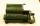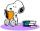# Equatiom

Solve equation with negatives:

X/(-5) + 2 = -9

X =  55

### Step-by-step explanation:

X/(-5) + 2 = -9

0.2X = 11

X = 55

Our simple equation calculator calculates it.Did you find an error or inaccuracy? Feel free to write us. Thank you!Tips to related online calculators
Do you have a linear equation or system of equations and looking for its solution? Or do you have a quadratic equation?

## Related math problems and questions:

• Evaluate expression 2Evaluate expression with negatives: (-3)+4+(-8)+(-6)+4+(-1)
• Multiplication negativesJosh says that (4)(-7)(-1) is equal to (4)(7). Evangelina says (4)(-7)(-1) is equal to (-28)(-1) . Who is correct? Explain your reasoning.
• Equation 11Solve equation: 0=y-1,2.(y-1,5)
• Find xSolve: if 2(x-1)=14, then x= (solve an equation with one unknown)
• IntegersMay be the sum of two integers less than their difference?
• Diofant equationIn the set of integers (Z) solve the equation: 212x +316y =0 Write result with integer parameter t in Z (parameter t = ...-2,-1,0,1,2,3... if equation has infinitely many solutions)
• Equation 25Solve following simple equation: 3/4(x+5)=1/2(x+9)
• Equation 15Solve equation with variables on both sides:
• Equation algebraogramSolve the equation: oco + ivo = cita How much has the task of solutions?
• Simple equation 5Solve equation with fractions: X × 3/8 = 1/2
• Simple equation 324 = n • 27, solve for n
• EquationSolve equation and check the result: 1.4x - 3/2 + x - 9,8 = x + 0,4/3 - 7 + 1,6/6
• Equation 29Solve next equation: 2 ( 2x + 3 ) = 8 ( 1 - x) -5 ( x -2 )
• Simple equation 9Solve the following equation: -8y+5=-9y+9
• Simple equation 8Solve the following equation: 36=-(1+7x)-6(-7-x)
• Equation with one variableSolve the following equation with one unknown: 5(7s + 5) =130
• Two numbers 6Fill two natural numbers a, b: 7 + blank- blank = 5